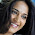## Python Iterator

##### Share it Please
Generally Iteration is a term for taking each item of something, one by one. Any time we use a loop, explicit or implicit to go over a group of items we are doing iteration.

Both iterable and Iterator has different meaning. An Iterable is an object that has an __Iter__ method defined which will return a Iterator. So an iterable is an object that you can get an iterator from.

An iterator is an object with a next (Python 2) or __next__ (Python 3) method.
Whenever you use a for loop, or map, or a list comprehension, etc. in Python, the next method is called automatically to get each item from the iterator, thus going through the process of iteration.

In this article we will see how we can define a Iterator. This article is based on Python 2.7 and hence we define a next() method ( where are __next__ in Python 3).

The Basic Iterator Example looks like this,

>>> s = "cat” # s is an ITERABLE
# s is a str object that is immutable

Here s is an ITERABLE and a String object that is immutable

>>> t = iter(s)

In the above case  t is an ITERATOR which has a state pointing from “c” in “cat”. The t has an next() method and an __iter__() method defined.

>>> t.next()
'c'
>>> t.next()
'a'
>>> t.next()
't'
>>> t.next()
Traceback (most recent call last):
File "<stdin>", line 1, in <module>
StopIteration

We can call the next() method on the Iterator “t” until to the end. Once the end is reached, the StopIteration is thrown

Now lets see how we can define our own Iterable class which will give us a Iterator when used

class Reverse:
def __init__(self,data):
self.data = data
self.index = len(data)

def __iter__(self):
return self

def next(self):
if self.index==0:
raise StopIteration
self.index = self.index-1
return self.data[self.index]

def Main():
ref = Reverse("This is Jagadish")
for c in ref:
print c

if __name__  == "__main__":
Main()

When you  run the code, we see
[djas999@vx181d imp]\$ python hello.py
h
s
i
***

Lets check the code. As we said an Iterator is obtained from an Object that has the __iter__ and next() ( __next__() in Python 3) defined. In the above, we can see that we have written a Class Reverse which will give us the reverse of the String that we pass. The class defines a __iter__() and next() methods. The __iter__() return the object itself where as the next() method defined returns elements one by one in reverse order. We also made sure to throw an Stop Iteration exception when the string passed is null or the elements reached to the end.

Thus I hope this will give the basic understating of the Iterator in Python

#### 1 comment :

1.I like your blog, I read this blog please update more content on python, further check it once at python online course